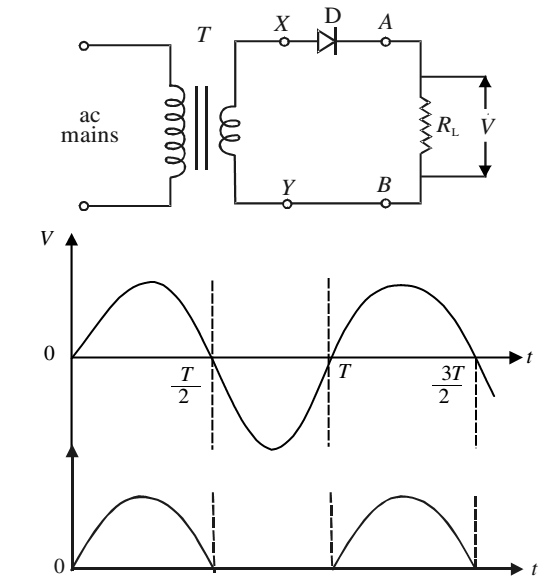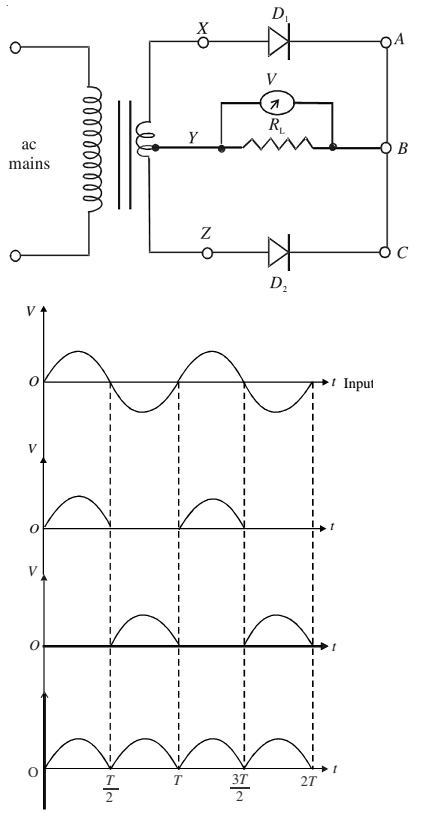# p-n Junction Diode as Rectifier

The electricity supply in homes provides ac voltage. It is a sinusoidal signal of frequency 50 Hz . It means that voltage (or current) becomes zero twice in one cycle, i.e., the waveform has one positive and other negative half cycle varying symmetrically around zero voltage level. The average voltage of such a wave is zero.

### Half-Wave Rectification

The signal from ac mains is fed into a step down transformer T which makes it available at the terminals X and Y. The load resistance RL is connected to these terminals through a p-n junction diode D.

The potential at terminal X with respect to Y will vary as a sine function with time. In the positive half cycle, during the time interval 0 to T/2, diode D will be forward biased and conduct, i.e., current flows through RL from A to B. However, during the negative half cycle, i.e., in the interval T/2 to T, D is reverse biased and the junction will not conduct, i.e. no current flows through RL.Since the p-n junction conducts only in one-half cycle of the sine wave, it acts as a half-wave rectifier.

During the non-conducting half cycle, the maximum reverse voltage appearing across the diode is equal to the peak ac voltage Vm. The maximum reverse voltage that a diode can oppose without breakdown is called its Peak Inverse Voltage (PIV). For rectification, you must choose a diode having PIV greater than the peak ac voltage to be rectified by it, otherwise it will get damaged.

The dc voltage, Vdc across RL, as measured by voltmeter in case of half-wave rectifier, is given by

Vdc = Vm

The dc current Idc through the load resistance RL is given by

Idc = Vdc/RL

### Full-Wave Rectification

For full-wave rectification, feed the input signal in a centre tapped step down transformer. It has two identical secondary winding connected in series.

D1 and D2 are two p-n junction diodes. One end of the load resistance RL is connected to the central point Y of the secondary windings and the other end is connected to the cathode terminals of the diodes D1 and D2. The anodes of these diodes are connected respectively to the ends X and Z of the secondary winding.

The potentials at the ends X and Z are in opposite phase with respect to Y, i.e., when potential of X is positive, Z will be negative and vice versa.First terminal X is positive and Z is negative with respect to Y. In this condition, diode D1 will conduct but D2 will not conduct. The current will flow through the load from B to Y. During the next half cycle, terminal X will be negative and Z will be positive. Under this condition, diode D2 conducts and current will again pass through the load resistance in the same direction, that is from B to Y.

Since current through the load now flows over the entire cycle of the sine wave, this is called full-wave rectification.

The dc voltage Vdc and dc current Idc are given by

Vdc = 2 × Vm

Idc = Vdc/RL

The unidirectional current flowing through the load resistance after full-wave rectification pulsates from maximum to minimum (zero) and is not useful for any practical application. To reduce the fluctuating component and obtain more steady current, the pulsating part is filtered.

### Filtering

Impedance offered by a capacitor to the flow of ac depends on its frequency. Therefore, a capacitor connected across the load resistance, filters out high frequency component.

The capacitor gets charged to nearly maximum potential Vm when diode D1 conducts for period t = T/4. When the current tends to decrease for T/4 < t < T/2, the capacitor discharges itself and tries to maintain current through the load, reducing fluctuations considerably.The larger the value of capacitor and the load resistance, the lower will be the fluctuations in the rectified dc. The capacitor connected across the load to reduce fluctuations is called a filter capacitor.